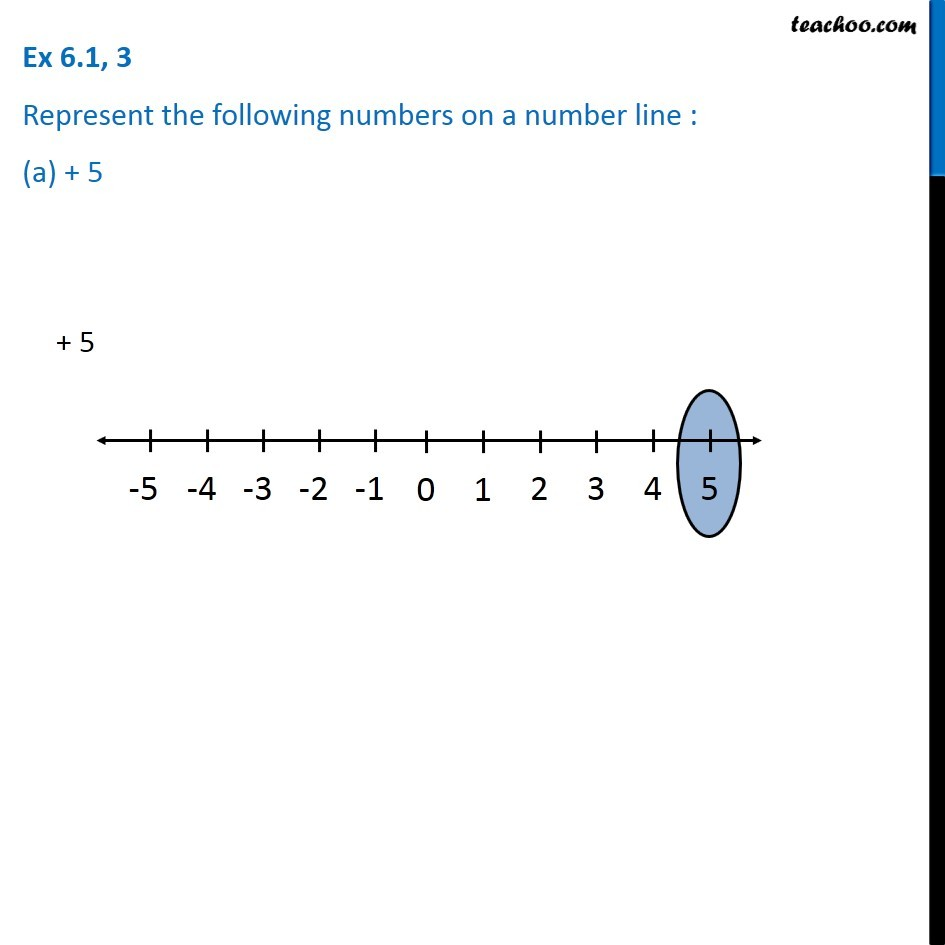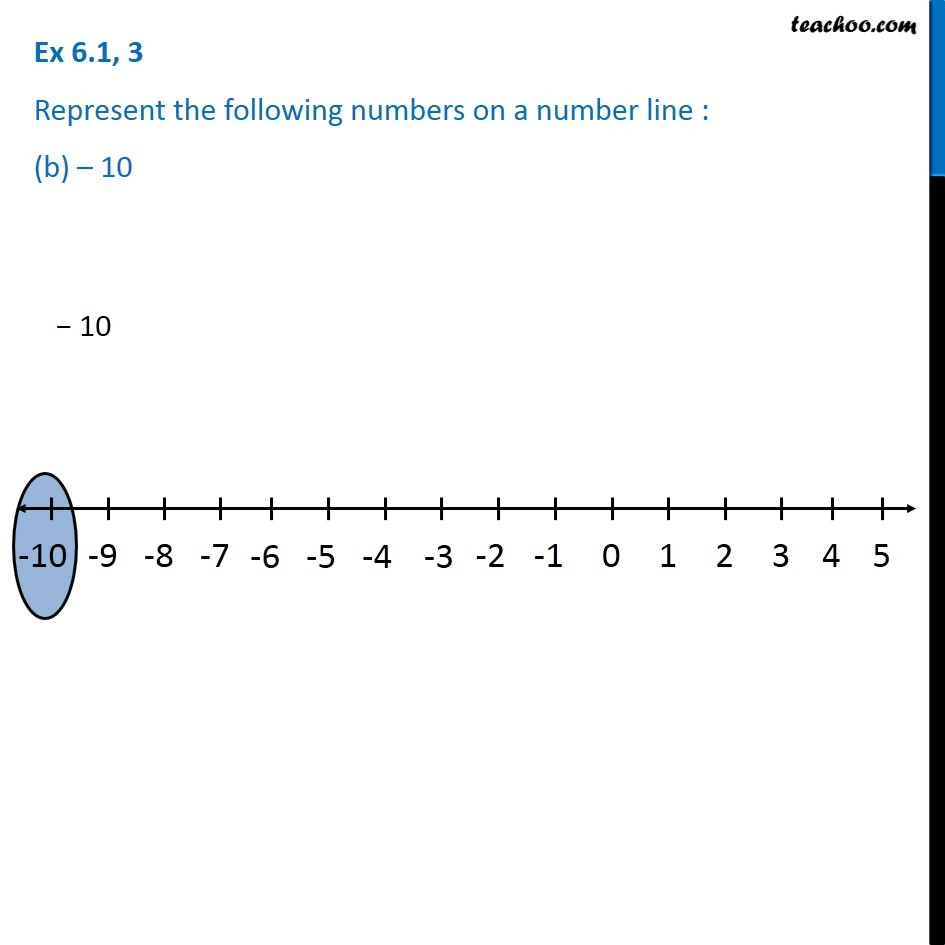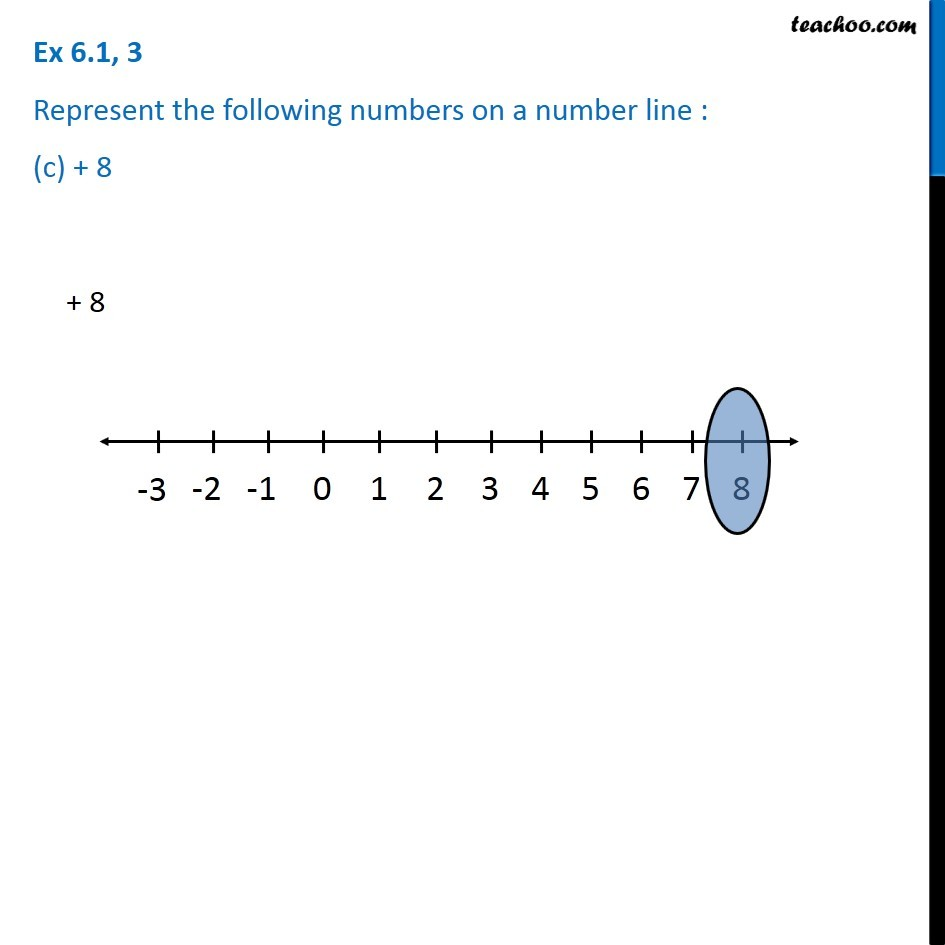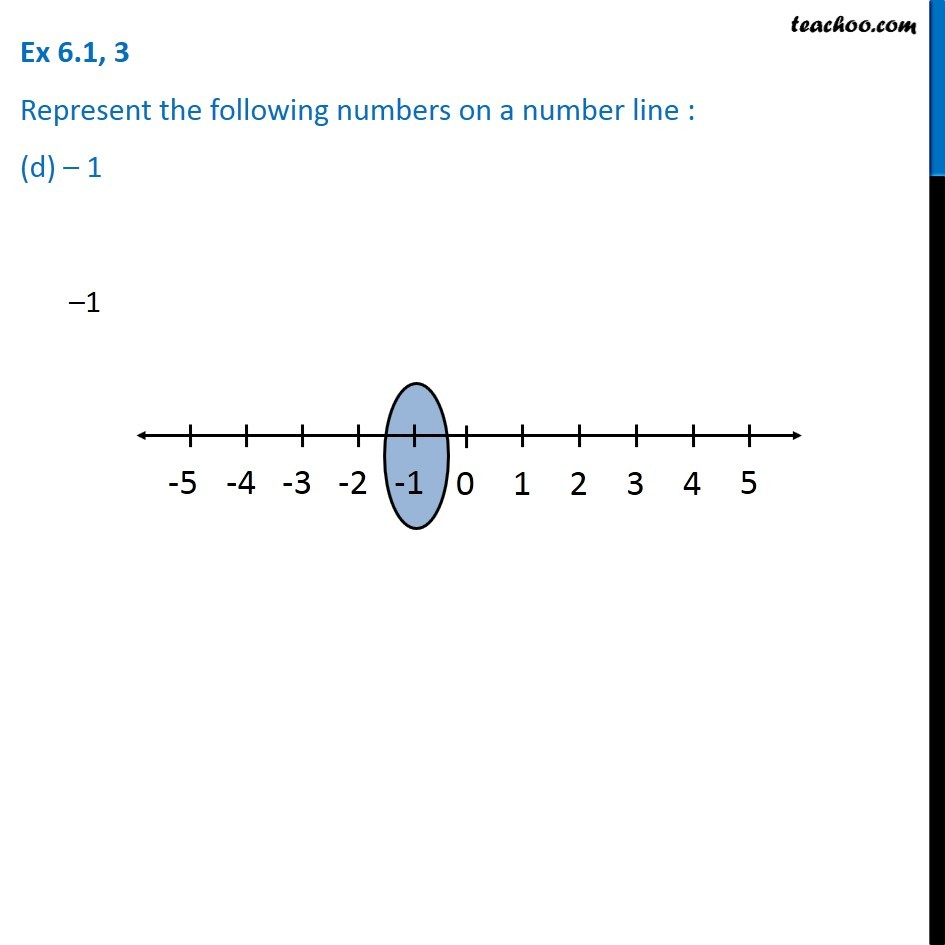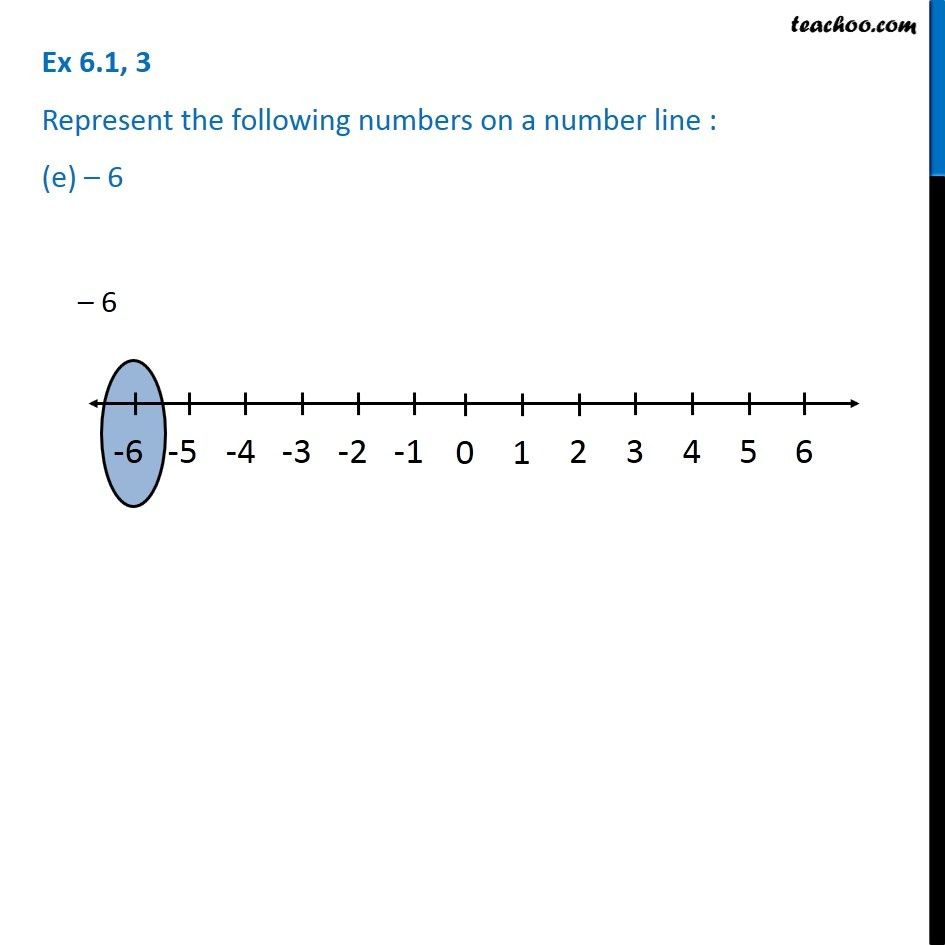1. Chapter 6 Class 6 Integers
2. Serial order wise
3. Ex 6.1

Transcript

Ex 6.1, 3 Represent the following numbers on a number line : (a) + 5 (b) -10 (c) +8 (d) -1 (e) -6 For +5 We follow these steps 1. Draw a line 2. In the middle, write 0 3. On the right of 0, we have positive numbers. Mark 1, 2, 3, 4, 5 with distance between 0 and 1 same as distance between 1 and 2 and so on... 4. Similarly, on the left of 0, we have negative numbers. Mark -1, -2, -3, -4, -5 on the left of number line. Mark +5 on the number line. Similarly, for +10, we make number line from -10 to +5. and so on

Ex 6.1